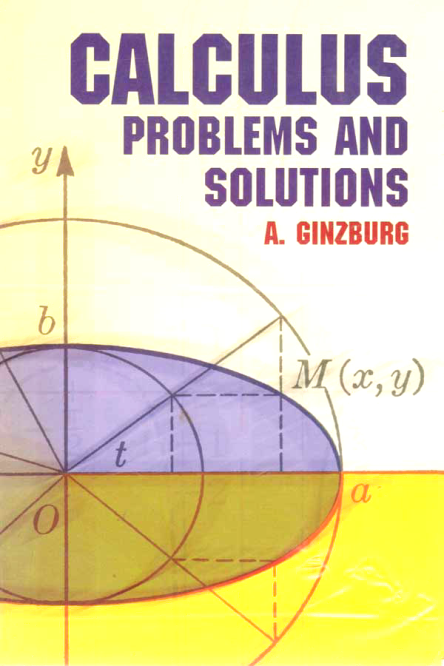## martes, 23 de agosto de 2016

### Categoría: Cálculo Calculus Problems and Solutions – A. GinzburgThis is the first of a series of problem books in analysis, analytic geometry, and higher algebra. The main purpose of this series is to provide the student with a rich collection of carefully selected material designed to increase his understanding and skill in handling problems in the above fields. To this end a large number of problems are presented and their solutions are given in full detail. To improve understanding, some prob­lems of more difficult character are included, the solution of which requires deeper insight in the topics treated.

More than 1200 problems are presented in this book. Some of these were taken from examination papers of the Technion, Israel Institute of Technology. Others were drawn from various sources and there seems to be no point in endeavoring to trace their origins.

The order of exposition adopted here seems quite natural; however, care has been taken so that the book may be used in a course in which the topics are arranged differently.

Every section begins with a brief explanation of the basic notions and theorems to be used. In general, the theorems are given without proof. The main part of every section is devoted to problems, a large number of which are immediately followed by solutions; others have solutions to be found only at the end of the book. It is believed that this will encour­age the reader to solve the problem by himself and only afterwards to look for the printed solution. The comparison of solutions will often be beneficial, as it will afford a check on the work and, occasionally, encounters with new methods.

Some problems, of course, have a variety of solutions and it may easily happen that the one given here is not the simplest possible. Remarks in this and other regards will be welcomed by the author.

My thanks are due to Dr. M. Edelstein, who read the manuscript and made many valuable suggestions, and to Dr. Emory P. Starke who super­vised the production of the book.

Contents:

I: SEQUENCES
1.1. Basic definitions and theorems
1.2. Examples and exercises on general notions
1.3. Representation of a number by sequences
1.4. Evaluation of N(e)
1.5. Sequences given in the form n«+i «
1.6. Methods for the evaluation of limits

II: FUNCTIONS OF A SINGLE VARIABLE
2.1. Definition and notation
2.2. The elementary functions
2.3. Domain of definition
2.4. Even and odd functions
2.5. Rational functions
2.6. Logarithmic functions
2.7. Trigonometric functions
2.8. Hyperbolic functions
2.9. Inverse functions
2.10. The inverse trigonometric functions
2.11. The inverse hyperbolic functions
2.12. Composite functions
2.13. Periodic functions

III: LIMIT OF A FUNCTION
3.1. Definitions and general exercises
3.2. Evaluation of limits
3.3. Continuity

IV: DIFFERENTIAL CALCULUS FOR FUNCTIONS OF A SINGLE VARIABLE
4.1. The notion of derivative and its physical and geometric interpretation
4.2. Evaluating derivatives
4.3. Evaluating derivatives of explicit functions
4.4. Differentiation of implicit functions
4.5. Parametric differentiation
4.6. Special eases in calculating derivatives
4.7. Higher derivatives
4.8. Calculation of t/
4.9. Graphical differentiation
4.10. Various examples

V: FUNDAMENTAL THEOREMS OF THE DIFFERENTIAL CALCULUS
5.1. The theorems of Rolle, Lagrange, and Cauchy
5.2. Taylor’s and Maclaurin’s formulas
5.3. Indeterminate forms: L’Hdpital’s rule

VI: APPLICATIONS OF DIFFERENTIAL CALCULUS
6.1. Rate of change
6.2. Locating intervals in which a function increases or decreases
6.3. Minima and maxima
6.4. Concavity: points of inflection
6.5. Asymptotes
6.6. Curve tracing
6.7. Graphs in polar coordinates
6.8. Parametric equations
6.9. Tangent and normal
6.10. The order of contact
6.11. Osculating circle, radius of curvature
6.12. Evolute and involute
6.13. Solution of equations by Newton’s approximation method

VII: THE DIFFERENTIAL
7.1. Definition of the differential
7.2. The invariance of the form of the differential
7.3. The differential as the principal part of the increment of the function: application to approximate calculations
7.4. Higher order differentials

VIII: THE INDEFINITE INTEGRAL
8.1. Definition and basic properties
8.2. Immediate integrals
8.3. The method of substitution
8.4. Integration by parts
8.5. Integrals of rational functions
8.6. Irrational integrals
8.7. Trigonometric integrals
8.8. Integrals of exponential and hyperbolic functions
8.9. Miscellaneous integrals

IX: THE DEFINITE INTEGRAL
9.1. Definition
9.2. Basic properties of the definite integral
9.3. Evaluation of the definite integral from its definition
9.4. Estimation of definite integrals
9.5. The mean value theorem of integral calculus
9.6. Integrals with variable limits
9.7. Evaluation of definite integrals
9.8. Changing the variable of integration
9.9. Approximate integration
9.10. Improper integrals
9.11. Miscellaneous problems

X: APPLICATIONS OF THE DEFINITE INTEGRAL
10.1. Computation of plane areas
10.2. Computation of arc length
10.3. Computation of volumes
10.4. Area of a surface of revolution
10.5. Moment of mass: centroids
10.6. Pappus’ theorems
10.7. Moment of inertia
10.8. Physics problems

XI: INFINITE SERIES
11.1. The general notion of a number series
11.2. Convergence of series with positive terms
11.3. Convergence of series with positive and negative terms
11.4. Arithmetic operations on series
11.5. Series of functions
11.6. Power series: radius of convergence
11.7. Taylor’s and Maclaurin’s scries: operations on power series
11.8. Applications of Taylor’s and Maclaurin’s expansions

XII: VARIOUS PROBLEMS PART TWO; SOLUTIONS, HINTS, ANSWERS INDEX
LIST OF GREEK LETTERS

Contraseña: www.freelibros.org